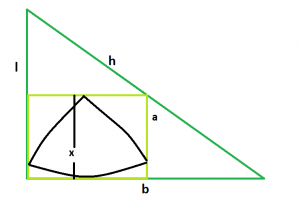Related Articles
Biggest Reuleaux Triangle within a Square which is inscribed within a Right angle Triangle
• Last Updated : 16 Mar, 2021

Given here is a right angle triangle with height l, base b & hypotenuse h, which inscribes a square which in turn inscribes a reuleaux triangle. The task is to find the maximum possible area of this reuleaux triangle.
Examples:

```Input: l = 5, b = 12, h = 13
Output: 8.77914

Input: l = 3, b = 4, h = 5
Output: 2.07116```Approach: We know, the side of the square inscribed within a right angled triangle is, a = (l*b)/(l+b), please refer Area of a largest square fit in a right angle triangle
Also, in the reuleaux triangle, x = a
So, x = (l*b)/(l+b)
So, Area of the Reuleaux Triangle is, A = 0.70477*x^2 = 0.70477*((l*b)/(l+b))^2.
Below is the implementation of the above approach:

## C++

 `// C++ Program to find the biggest Reuleaux triangle``// inscribed within in a square which in turn``// is inscribed within a circle``#include ``using` `namespace` `std;` `// Function to find the biggest reuleaux triangle``float` `Area(``float` `l, ``float` `b, ``float` `h)``{` `    ``// the height or base or hypotenuse``    ``// cannot be negative``    ``if` `(l < 0 || b < 0 || h < 0)``        ``return` `-1;` `    ``// height of the reuleaux triangle``    ``float` `x = (l * b) / (l + b);` `    ``// area of the reuleaux triangle``    ``float` `A = 0.70477 * ``pow``(x, 2);` `    ``return` `A;``}` `// Driver code``int` `main()``{``    ``float` `l = 5, b = 12, h = 13;``    ``cout << Area(l, b, h) << endl;` `    ``return` `0;``}`

## Java

 `// Java Program to find the biggest Reuleaux triangle``// inscribed within in a square which in turn``// is inscribed within a circle``import` `java.util.*;``import` `java.text.DecimalFormat;` `class` `GFG``{` `// Function to find the biggest reuleaux triangle``static` `double` `Area(``double` `l, ``double` `b, ``double` `h)``{` `    ``// the height or base or hypotenuse``    ``// cannot be negative``    ``if` `(l < ``0` `|| b < ``0` `|| h < ``0``)``        ``return` `-``1``;` `    ``// height of the reuleaux triangle``    ``double` `x = (l * b) / (l + b);` `    ``// area of the reuleaux triangle``    ``double` `A = ``0.70477` `* Math.pow(x, ``2``);` `    ``return` `A;``}` `// Driver code``public` `static` `void` `main(String args[])``{``    ``double` `l = ``5``, b = ``12``, h = ``13``;``    ``DecimalFormat df = ``new` `DecimalFormat(``"#,###,##0.00000"``);``    ``System.out.println(df.format(Area(l, b, h)));``}``}` `// This code is contributed by``// Shashank_Sharma`

## Python3

 `# Python3 Program to find the biggest``# Reuleaux triangle inscribed within``# in a square which in turn is inscribed``# within a circle``import` `math as mt` `# Function to find the biggest``# reuleaux triangle``def` `Area(l, b, h):` `    ``# the height or base or hypotenuse``    ``# cannot be negative``    ``if` `(l < ``0` `or` `b < ``0` `or` `h < ``0``):``        ``return` `-``1` `    ``# height of the reuleaux triangle``    ``x ``=` `(l ``*` `b) ``/``(l ``+` `b)` `    ``# area of the reuleaux triangle``    ``A ``=` `0.70477` `*` `pow``(x, ``2``)` `    ``return` `A` `# Driver code``l, b, h ``=` `5``, ``12``, ``13``print``(Area(l, b, h))` `# This code is contributed by``# Mohit kumar 29`

## C#

 `// C# Program to find the biggest Reuleaux triangle``// inscribed within in a square which in turn``// is inscribed within a circle``using` `System;` `class` `GFG``{` `// Function to find the biggest reuleaux triangle``static` `double` `Area(``double` `l, ``double` `b, ``double` `h)``{` `    ``// the height or base or hypotenuse``    ``// cannot be negative``    ``if` `(l < 0 || b < 0 || h < 0)``        ``return` `-1;` `    ``// height of the reuleaux triangle``    ``double` `x = (l * b) / (l + b);` `    ``// area of the reuleaux triangle``    ``double` `A = 0.70477 * Math.Pow(x, 2);` `    ``return` `A;``}` `// Driver code``public` `static` `void` `Main()``{``    ``double` `l = 5, b = 12, h = 13;``    ``Console.WriteLine((Area(l, b, h)));``}``}` `// This code is contributed by``// Mukul Singh`

## PHP

 ``

## Javascript

 ``
Output:
`8.77914`

Want to learn from the best curated videos and practice problems, check out the C++ Foundation Course for Basic to Advanced C++ and C++ STL Course for foundation plus STL.

My Personal Notes arrow_drop_up